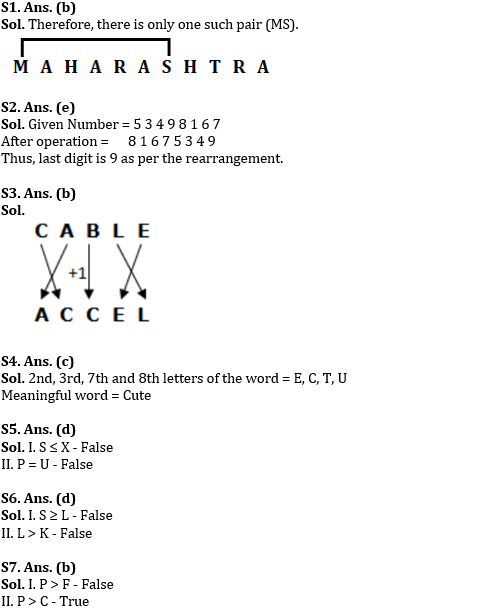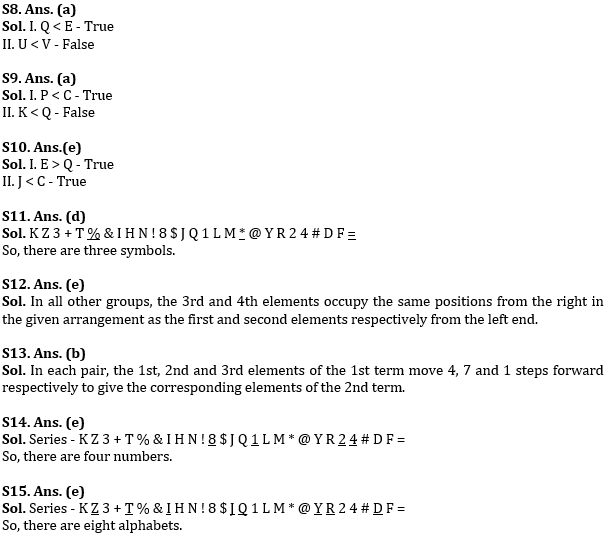Latest Banking jobs   »

# Reasoning Ability Quiz For SBI Clerk Prelims 2022- 27th May

Q1. How many such pairs of letters are there in the word ‘MAHARASHTRA’ each of which has as many letters between them in the word as in the English alphabet (Both forward and backward direction)?
(a) None
(b) One
(c) Two
(d) Three
(e) More than three

Q2. The positions of the first and the fifth digits in the number 53498167 are interchanged. Similarly, the positions of the second and the sixth digits are interchanged and so on. Which of the following will be the last digit after the rearrangement?
(a) 7
(b) 5
(c) 3
(d) 4
(e) None of these

Q3. In a particular code language, ‘CLAIM’ is coded as ‘LCBMI’, so how will ‘CABLE’ be coded in the same code language?
(a) ECCAL
(b) ACCEL
(c) CCALE
(d) LECCA
(e) None of the above

Q4. How many meaningful word/words can be formed using second, third, seventh and eighth letters of the word RECAPITULATE using each letter only once in each word?
(a) None
(b) One
(c) Two
(d) Three
(e) None of these

Directions (5-10): In each of the following questions, assuming the given statements to be true, find which of the following options holds true:

Q5. Statements: P = X ≥ W > Y, S ≤ T < P, Y = U
Conclusions:
I. S ≤ X
II. P = U
(a) Only I is true
(b) Only II is true
(c) Either I or II is true
(d) Neither I nor II is true
(e) Both I and II are true

Q6. Statements: S ≥ Q, P < K, Q ≤ L, P = L
Conclusions:
I. S ≥ L
II. L > K
(a) Only I is true
(b) Only II is true
(c) Either I or II is true
(d) Neither I nor II is true
(e) Both I and II are true

Q7. Statements: F ≥ T, A ≤ C, P ≥ T, T > C
Conclusions:
I. P > F
II. P > C
(a) Only I is true
(b) Only II is true
(c) Either I or II is true
(d) Neither I nor II is true
(e) Both I and II are true

Q8. Statements: J ≤ K, U > Q < T, E ≥ U, E = V
Conclusions:
I. Q < E
II. U < V
(a) Only I is true
(b) Only II is true
(c) Either I or II is true
(d) Neither I nor II is true
(e) Both I and II are true

Q9. Statements: Q ≥ S, S < K, C = K, P < S
Conclusions:
I. P < C
II. K < Q
(a) Only I is true
(b) Only II is true
(c) Either I or II is true
(d) Neither I nor II is true
(e) Both I and II are true

Q10. Statements: J < H = F, C ≥ E > F, J > L ≥ Q
Conclusions:
I. E > Q
II. J < C
(a) Only I is true
(b) Only II is true
(c) Either I or II is true
(d) Neither I nor II is true
(e) Both I and II are true

Directions (11-15): Read the following arrangement carefully to answer the following questions.

K Z 3 + T % & I H N ! 8 \$ J Q 1 L M * @ Y R 2 4 # D F =

Q11. How many such symbols are there in the above arrangement each of which is either immediately followed by a number or immediately preceded by a letter, but not both?
(a) None
(b) One
(c) Two
(d) Three
(e) More than three

Q12. Four of the following five are alike in a certain way. Which one does not belong to the group?
(a) 3 Z D F
(b) % T 2 4
(c) K T = 4
(d) % I 2 Y
(e) + # @ Q

Q13. If 3 Z T is related to & H % in the same way, Q J L is to ____?
(a) Y R *
(b) * Y M
(c) @ * R
(d) @ Y L
(e) None of these

Q14. How many such numbers are there, each of which is either immediately followed by a symbol or immediately preceded by a letter, but not both?
(a) None
(b) One
(c) Two
(d) Three
(e) More than three

Q15. How many such letters are there, each of which is either immediately followed by a number or immediately preceded by a symbol but not both?
(a) None
(b) One
(c) Two
(d) Three
(e) More than three

Solutions#### Congratulations!